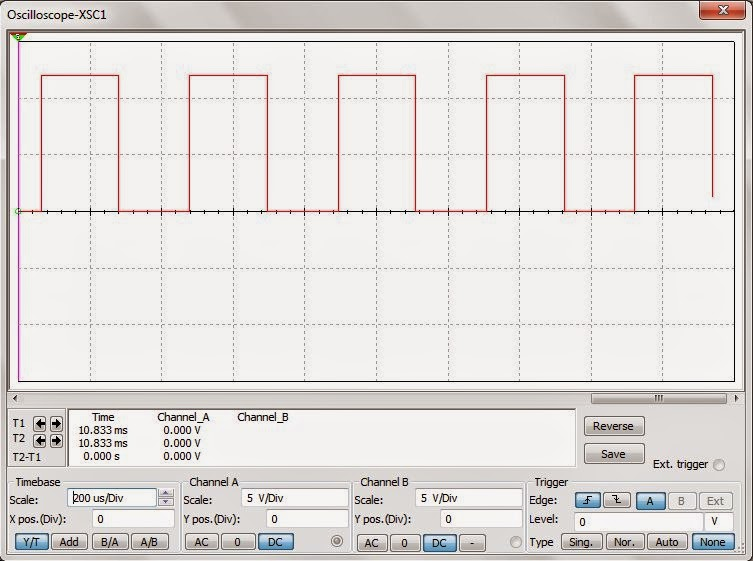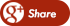﻿ Square wave generator?Back

## Square wave generator?

By Admin onSquare wave generator

Introduction

The circuit to the right uses a comparator with both positive and negative feedback to control its output voltage. Because the negative feedback path uses a capacitor while the positive feedback path does not, however, there is a time delay before the comparator is triggered to change state. As a result, the circuit oscillates, or keeps changing state back and forth at a predictable rate. Because no effort is made to limit the output voltage, it will switch from one extreme to the other. If we assume it starts at -10 volts, then the voltage at the "+" input will be set by R2 and R1 to a fixed voltage equal to -10R1/(R1 + R2) volts. This then becomes the reference voltage for the comparator, and the output will remain unchanged until the "-" input becomes more negative than this value. But the "-" input is connected to a capacitor (C) which is gradually charging in a negative direction through resistor Rf. Since C is charging towards -10 volts, but the reference voltage at the "+" input is necessarily smaller than the -10 volt limit, eventually the capacitor will charge to a voltage that exceeds the reference voltage. When that happens, the circuit will immediately change state. The output will become +10 volts and the reference voltage will abruptly become positive rather than negative. Now the capacitor will charge towards +10 volts, and the other half of the cycle will take place. The output frequency is given by the approximate equation:

 fout = 1 2RfC ln ( 2R1 + 1 ) R2

The non-sinusoidal waveform generators are also called relaxation oscillators. The op-amp relaxation oscillator shown in figure is a square wave generator. In general, square waves are relatively easy to produce. Like the UJT relaxation oscillator, the circuit’s frequency of oscillation is dependent on the charge and discharge of a capacitor C through feedback resistor R,. The “heart” of the oscillator is an inverting op-amp comparator The comparator uses positive feedback that increases the gain of the amplifier. In a comparator circuit this offer two advantages. First, the high gain causes the op-amp’s output to switch very quickly from one state to another and vice-versa. Second, the use of positive feedback gives the circuit hysteresis. In the op-amp square-wave generator circuit given in figure, the output voltage vout is shunted to ground by two Zener diodes Z1 and Z2 connected back-to-back and is limited to either VZ 2 or –VZ 1. A fraction of the output is fedback to the non-inverting (+) input terminal.Combination of IL and C acting as a low-pass R-C circuit is used to integrate the output voltage vout and the capacitor voltage vc is applied to the inverting input terminal in place of external signal. The differential input voltage is given as vin = vc - β vout When vin is positive, vout = – Vz1 and when vin is negative vout = + Vz 2. Consider an instant of time when vin < 0. At this instant vout = + Vz 2 , and the voltage at the non-inverting (+) input terminal is β Vz 2 , the capacitor C charges exponentially towards Vz 2, with a time constant Rf C. The output voltage remains constant at Vz 2 until vc equal β Vz 2.

Working  of square wave generator

This multi-vibrator works in same way as a comparator by comparing the two input voltages and giving the output signals regarding the input voltage values. When the circuit is switched ON the C1 starts charging through the Resistors R1 and R2.The Resistor R2 was a variable one and it was used to change the frequency range of the output signal. When the voltage across the Capacitor rises above the junction R3 and R4 the output switches to fully negative voltage.C1 will begin to discharge and charges in the opposite direction. When the negative voltage across the C1 falls below that pin 3 the circuit switches back quickly to the fully positive output value thereby giving out the high signal. The above cycle repeats endlessly resulting in giving out a series of square wave pulses as output through the Pin 3.The value of capacitor should be around 1uf and this circuit is capable of giving out output signal of frequency of (1Hz-25Hz).This circuit will be a great alternative for other Astable multi-vibrator circuits and cheap one too.Square wave Oscillator

A number of operational amplifier circuits are provided here that are set up to be square wave oscillators. A square wave is a period wave pulse train with a 50 percent duty cycle. The operational amplifier is really just a high-gain amplifier, so oscillation is not a problem with just a little positive feedback [R4 provides the positive feedback in the first schematic]. The exponential voltage which develops across the capacitor is converted to a current by resistor R1. The rising and falling exponential voltage is set by the product of the resistor capacitor combination. The frequency of oscillation is determined by the following equation: F = 0.7 / (R1 x C). The period would then be 1.4*(R1 x C). Because only one timing path exits, the capacitor changes and discharges though the same resistor, the circuit produces a square wave. the trip points, when the oscillator switches, is set by the comparator input which is determined by V+, R2 and R4. In most cases R2 is made equal to R4.FOR MORE DETAILS CLICK HERE